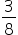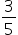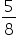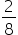Mathematics
Easy

Question

# From a bag of 3 red and 5 blue balls, a ball is drawn randomly. The probability of getting blue is

##Hint:

## The correct answer is:### Here, there are 3 red balls and 5 blue balls in a bag. We have to find the probability of getting a blue balls.Total number of outcomes = Number of red balls + Number of blue balls= 3 + 5= 8Number of favourable outcomes = Number of blue balls = 5Therefore, P ( getting a blue ball) = 5/8Hence, the correct option is (c).

0 ≤ P(E) ≤ 1.Also sum of probability of all events is 1 i.e  Here, P(red) + P(blue) = 1#### With Turito Foundation.#### Get an Expert Advice From Turito.